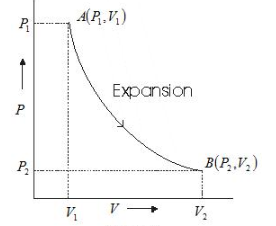# Work done in an Isothermal Process

## Work done in Isothermal process

• In an isothermal process temperature remains constant.
• Consider pressure and volume of ideal gas changes from (P1, V1) to (P2, V2).At any intermediate stage with pressure P and volume change from V to $V + \Delta V$ ($\Delta V$ small)
then from first law of thermodynamics
$\Delta W = P \Delta V$
Now taking $\Delta V$ approaching zero i.e. $\Delta V \rightarrow 0$ and summing $\Delta W$ over entire process we get total work done by gas so we have
$W = \int_{V_1}^{V_2} PdV$
where limits of integration goes from V1 to V2
as $PV = nRT$ we have $P = \frac {nRT}{V}$
Therefore $W = \int_{V_1}^{V_2} (\frac {nRT}{V}) dV$
On integrating we get,
$W=nRT ln (\frac {V_2}{V_1})$
Where n is number of Moles in sample of gas taken.
and $ln= log_e$
• This can be also written as
$W=2.303 nRT log (\frac {V_2}{V_1})$
• This can also be expresses in terms of Initial Pressure and Final Pressure also $W=2.303 nRT log (\frac {P_1}{P_2})$
• We can easily conclude from Work done equation ,for $V_2 > V_1$, W > 0 and for $V_2 < V_1$, W < 0. That is, in an isothermal expansion, the gas absorbs heat and does work while in an isothermal compression, work is done on the gas by the environment and heat is released.

## Solved Example for Work done in a Isothermal Process

Question 1
2 moles of an ideal is isothermally expanded to 3 times its original volume at 300K . Calculate the Work done and heat absorbed by the gas?
Given ( R=8.31 J/mol-K and $log_e 3=.4771$)
solution
Here n= 3, $V_2 =3 V_1$, T=300 K
Now using the above equation
$W=nRT ln (\frac {V_2}{V_1})$
Substituting the above values, we get
$W= 5.48 \times 10^3$ J
Now heat absorbed = Work done= $5.48 \times 10^3$ J = $1.31 \times 10^3$ C

Question 2
An ideal gas undergoes isothermal process from some initial state i to final state f. Choose the correct alternatives.
(a) dU = 0
(b) dQ= 0
(c) dQ = dU
(d) dQ = dW
solution
(a) and (d)